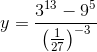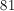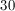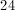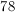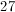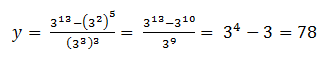## Example Questions

### Example Question #2191 : Act Math

Simplify the following expression:

4x2 * 5x3

20x5

9x6

5x5

20x6

9x5

20x5

Explanation:

Since you are multiplying, you multiply the integers and add the exponents, giving you 20x5.

### Example Question #12 : Exponential Operations

Find the product: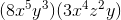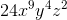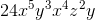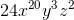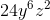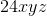Explanation:

Find the product:Multiply the coefficients, and use the rule for multiplying exponents (add them):### Example Question #21 : Exponential Operations

(b * b* b7)1/2/(b3 * bx) = b5

If b is not negative then x = ?

1

–1

–2

7

–2

Explanation:

Simplifying the equation gives b6/(b3+x) = b5.

In order to satisfy this case, x must be equal to –2.

### Example Question #14 : Exponential Operations

If〖7/8〗n= √(〖7/8〗5),then what is the value of n?

25

1/5

2/5

5/2

√5

5/2

Explanation:

7/8 is being raised to the 5th power and to the 1/2 power at the same time. We multiply these to find n.

### Example Question #1561 : Gre Quantitative Reasoning

Simplify: (x3 * 2x4 * 5y + 4y2 + 3y2)/y

10x7 + 7y

10x11 + 7y3

10x7 + 7y3

10x7y + 7y2

10x7 + 7y

Explanation:

Let's do each of these separately:

x3 * 2x4 * 5y = 2 * 5 * x* x* y = 10 * x7 * y = 10x7y

4y2 + 3y2 = 7y2

Now, rewrite what we have so far:

(10x7y + 7y2)/y

There are several options for reducing this.  Remember that when we divide, we can "distribute" the denominator through to each member.  That means we can rewrite this as:

(10x7y)/y + (7y2)/y

Subtract the y exponents values in each term to get:

10x7 + 7y

### Example Question #16 : Exponential Operations

Compare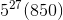and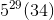.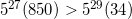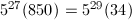The answer cannot be determined from the information given.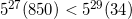Explanation:

To compare these expressions more easily, we'll change the first expression to have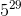in front. We'll do this by factoring out 25 (that is,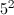) from 850, then using the fact that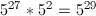.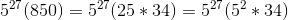When we combine like terms, we can see that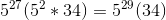. The two terms are therefore both equal to the same value.

### Example Question #21 : Exponents

Which of the following is equal to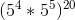?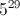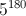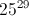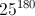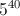Explanation: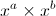is always equal to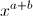; therefore, 5 raised to 4 times 5 raised to 5 must equal 5 raised to 9.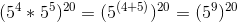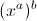is always equal to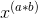. Therefore, 5 raised to 9, raised to 20 must equal 5 raised to 180.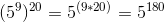### Example Question #62 : Exponents

Which of the following is equal to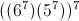?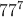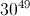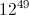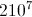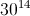Explanation:

First, multiply inside the parentheses: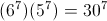.

Then raise to the 7th power: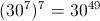.

### Example Question #21 : Exponents

Simplify: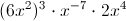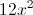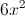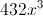Explanation:

Remember, we add exponents when their bases are multiplied, and multiply exponents when one is raised to the power of another. Negative exponents flip to the denominator (presuming they originally appear in the numerator).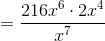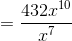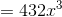### Example Question #13 : How To Multiply Exponents

Evaluate: